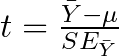# Parametric tests

Comparing means

This week we will be discussing how to compare between the means of normally distributed populations using population parameters (μ and σ). We call these tests parametric tests.

## T-distribution

In real-life studies, however, we rarely know the population standard deviation (σ).

We need to estimate it using the standard error of the mean (SE). This then changes our normal sampling distribution to a t-distribution.## T-critical value

The critical value of a t-distribution is combined 5% area under the tails of the distribution (2.5% on both sides). Only 2.5% of one tail is considered for a one-tailed test.

## 95% critical value for the mean## T-test suite

One-sample t-test: compare the mean of a sample to a proposed population mean.

Assumptions:

• Data is a random sample from the population
• Y is normally distributed in the populationPaired t-test: compare the mean difference between two samples where samples are paired  with each other (e.g., before and after observations from the each individual in a sample)

Assumptions:

• Data is a random sample from the population
• Paired differences normally distributed in the populationWe can also compare the means of two samples that are not paired, most comparisons of means will fall under the two-sample t-test.

Assumptions:

• Data is a random sample from the population
• Data is normally distributed in the population
• Variance between samples is equal## Checking normality

Checking normality is relatively straight forward. We produce a histogram of our observations and use the eyeball test. If it looks normal (i.e., like a bell curve), then we can assume our data is normal.

There is also a hypothesis test called the Shapiro-Wilk test, which is a goodness-of-fit test for the normal distribution. A P-value > 0.05 indicates that the data is likely to be from a population that is normally distributed.

## Checking homogeneity of variances

While we can use the “eyeball test” to determine if our data is from a normal distribution. We can’t eyeball equal variances. Instead, we can use a hypothesis tests. One way is the F-test, the other is Levene’s test.